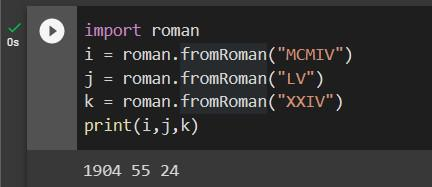GeeksforGeeks App
Open AppBrowser
Continue

# Python Program For Converting Roman Numerals To Decimal Lying Between 1 to 3999

Given a Roman numeral, the task is to find its corresponding decimal value.

Example :

```Input: IX
Output: 9
IX is a Roman symbol which represents 9

Input: XL
Output: 40
XL is a Roman symbol which represents 40

Input: MCMIV
Output: 1904
M is a thousand,
CM is nine hundred and
IV is four```

Roman numerals are based on the following symbols.

```SYMBOL       VALUE
I            1
IV           4
V            5
IX           9
X            10
XL           40
L            50
XC           90
C            100
CD           400
D            500
CM           900
M            1000```

Approach: A number in Roman Numerals is a string of these symbols written in descending order(e.g. M’s first, followed by D’s, etc.). However, in a few specific cases, to avoid four characters being repeated in succession(such as IIII or XXXX), subtractive notation is often used as follows:

• I placed before V or X indicates one less, so four is IV (one less than 5) and 9 is IX (one less than 10).
• X placed before L or C indicates ten less, so forty is XL (10 less than 50) and 90 is XC (ten less than a hundred).
• C placed before D or M indicates a hundred less, so four hundred is CD (a hundred less than five hundred) and nine hundred is CM (a hundred less than a thousand).

Algorithm to convert Roman Numerals to Integer Number:

1. Split the Roman Numeral string into Roman Symbols (character).
2. Convert each symbol of Roman Numerals into the value it represents.
3. Take symbol one by one from starting from index 0:
1. If current value of symbol is greater than or equal to the value of next symbol, then add this value to the running total.
2. else subtract this value by adding the value of next symbol to the running total.

Following is the implementation of the above algorithm:

## Python

 `# Python program to convert Roman``# Numerals to Numbers` `# This function returns value of``# each Roman symbol``def` `value(r):``    ``if` `(r ``=``=` `'I'``):``        ``return` `1``    ``if` `(r ``=``=` `'V'``):``        ``return` `5``    ``if` `(r ``=``=` `'X'``):``        ``return` `10``    ``if` `(r ``=``=` `'L'``):``        ``return` `50``    ``if` `(r ``=``=` `'C'``):``        ``return` `100``    ``if` `(r ``=``=` `'D'``):``        ``return` `500``    ``if` `(r ``=``=` `'M'``):``        ``return` `1000``    ``return` `-``1` `def` `romanToDecimal(``str``):``    ``res ``=` `0``    ``i ``=` `0` `    ``while` `(i < ``len``(``str``)):` `        ``# Getting value of symbol s[i]``        ``s1 ``=` `value(``str``[i])` `        ``if` `(i ``+` `1` `< ``len``(``str``)):` `            ``# Getting value of symbol s[i + 1]``            ``s2 ``=` `value(``str``[i ``+` `1``])` `            ``# Comparing both values``            ``if` `(s1 >``=` `s2):` `                ``# Value of current symbol is greater``                ``# or equal to the next symbol``                ``res ``=` `res ``+` `s1``                ``i ``=` `i ``+` `1``            ``else``:` `                ``# Value of current symbol is greater``                ``# or equal to the next symbol``                ``res ``=` `res ``+` `s2 ``-` `s1``                ``i ``=` `i ``+` `2``        ``else``:``            ``res ``=` `res ``+` `s1``            ``i ``=` `i ``+` `1` `    ``return` `res` `# Driver code``print``(``"Integer form of Roman Numeral is"``),``print``(romanToDecimal(``"MCMIV"``))`

Output:

`Integer form of Roman Numeral is 1904`

Complexity Analysis:

• Time Complexity: O(n), where n is the length of the string.
Only one traversal of the string is required.
• Space Complexity: O(1).
As no extra space is required.

Approach 2 – In this method, we will convert an integer to roman and back using an external module named roman. First, we must install it using the pip command. In the terminal, type the command below.

`!pip install roman`

Now after successful installation we will import the module and use the method fromRoman() which takes a Roman value as argument and converts it into decimal and returns it.

Syntax of fromRoman() method –

fromRoman(“<roman_value>”)

Inside the fromRoman method we need to pass the roman value as string. The value passed must be in uppercase letters.

Implementation –

## Python3

 `import` `roman``i ``=` `roman.fromRoman(``"MCMIV"``)``j ``=` `roman.fromRoman(``"LV"``)``k ``=` `roman.fromRoman(``"XXIV"``)``print``(i,j,k)`

Output –

`1904 55 24`Time Complexity – O(N)

Auxiliary Space – O(1)

Please refer complete article on Converting Roman Numerals to Decimal lying between 1 to 3999 for more details!

METHOD 3:Using re module

APPROACH:

This program takes a Roman Numeral as input and converts it to its equivalent Decimal value using a dictionary that maps the Roman Numerals to their corresponding values.

ALGORITHM:

1.Create a dictionary that maps each Roman Numeral to its corresponding Decimal value.
Initialize the result variable to zero.
2.Loop through the input Roman Numeral string.
3.Check if the value of the current Roman Numeral is greater than the previous Roman Numeral. If so, subtract twice the value of the previous Roman Numeral from the result.
4.Otherwise, add the value of the current Roman Numeral to the result.
5.Print the Decimal equivalent of the given Roman Numeral.

## Python3

 `# Python program to convert Roman Numerals to Decimal``import` `re``# Given input``roman_numeral ``=` `'MCMIV'` `# Creating a dictionary to store the Roman Numerals and their corresponding values``roman_dict ``=` `{``'I'``: ``1``, ``'V'``: ``5``, ``'X'``: ``10``, ``'L'``: ``50``, ``'C'``: ``100``, ``'D'``: ``500``, ``'M'``: ``1000``}` `# Initializing the result variable to zero``result ``=` `0` `# Looping through the Roman Numeral string and adding the corresponding value to the result variable``for` `i ``in` `range``(``len``(roman_numeral)):``    ``if` `i > ``0` `and` `roman_dict[roman_numeral[i]] > roman_dict[roman_numeral[i``-``1``]]:``        ``result ``+``=` `roman_dict[roman_numeral[i]] ``-` `2` `*` `roman_dict[roman_numeral[i``-``1``]]``    ``else``:``        ``result ``+``=` `roman_dict[roman_numeral[i]]` `# Printing the Decimal equivalent of the given Roman Numeral``print``(``"The Decimal equivalent of"``, roman_numeral, ``"is"``, result)`

Output

`The Decimal equivalent of MCMIV is 1904`

Time complexity:

The time complexity of the program is O(n), where n is the length of the input Roman Numeral string. This is because we loop through the string once to calculate the Decimal equivalent.

Auxiliary Space:

The space complexity of the program is O(1), because we only use a constant amount of extra space to store the dictionary and result variable.

My Personal Notes arrow_drop_up